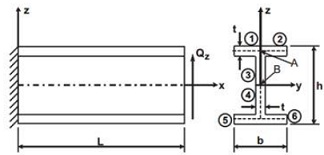Compute the bending stiffness of a cantilever i-beam

Solve the following problem:

Compute the torsional stiffness (GJR) of the beam in Example 1.

Example 1:

Compute the bending stiffness of a cantilever I-beam (Figure ) of length L = 30 cm, subjected to a tip-shear force Vz = 445 N. The dimensions of the beam are: h = 2.5 cm, b = h/2, t = h/16. The material is Kevlar-epoxy with the following elastic constants: E1 = 76.0 GP a, E2 = 5.56 GP a, G12 = 2.30 GP a, ν12 = 0.34. The lay-up sequence is [±30/0]S, six laminae (n = 6), with lamina thickness tk = t/6.